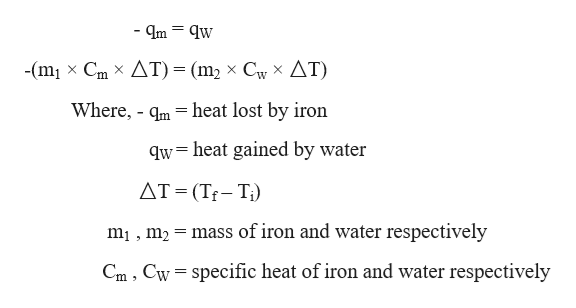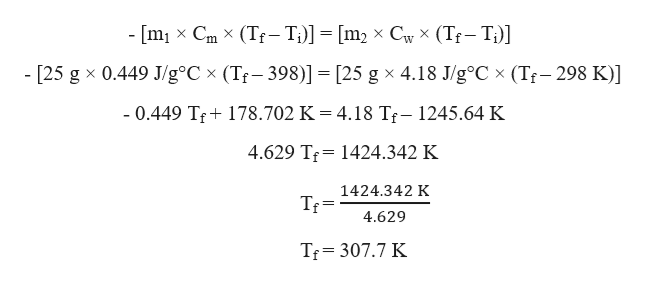# A piece of iron (mass = 25.0 g) at 398 K is placed in a styrofoam coffee cup containing 25.0 mL of water at 298 K. Assuming that no heat is lost to the cup or the surroundings, what will the final temperature of the water be? The specific heat capacity of iron = 0.449 J/g°C and water = 4.18J/g°C.

Question
2403 views
A piece of iron (mass = 25.0 g) at 398 K is placed in a styrofoam coffee cup containing 25.0 mL of water at 298 K. Assuming that no heat is lost to the cup or the surroundings, what will the final temperature of the water be? The specific heat capacity of iron = 0.449 J/g°C and water = 4.18
J/g°C.
check_circle

Step 1

Given,

Mass of iron (m1) = 25 g

Initial temperature of iron (Ti) = 398 K

Specific heat capacity of iron (Cm) = 0.449 J/g°C

Mass of water (m2) = 25 g

Initial temperature of water (Ti) = 298 K

Specific heat capacity of water (Cw) = 4.18 J/g°C

Step 2

Let the final temperature be Tf

Assuming that no heat is lost to the cup or the surroundings, therefore heat lost by iron will be equal to heat gained by water which can be written as:help_outlineImage Transcriptionclose- Im = qw -(m1 Cm X AT) (m2 x Cy X AT) Where, m heat lost by iron qw= heat gained by water AT (Tf-Ti) = mass of iron and water respectively mi, m2 Cw specific heat of iron and water respectively Cm fullscreen
Step 3

Substituting the given ...help_outlineImage TranscriptioncloseCwx (T T)] - [m x Cm X (Tr-T:)] [m2 X X - [25 g x 0.449 J/g°C x (Tr 398)] [25 g x 4.18 J/g°C x (Tr- 298 K)] X - 0.449 Tf 178.702 K = 4.18 Tf- 1245.64 K 4.629 T 1424.342 K 1424.342 K 4.629 Tf 307.7 K fullscreen

### Want to see the full answer?

See Solution

#### Want to see this answer and more?

Solutions are written by subject experts who are available 24/7. Questions are typically answered within 1 hour.*

See Solution
*Response times may vary by subject and question.
Tagged in

### Chemistry